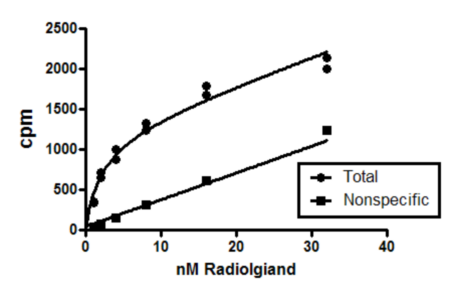## Please enable JavaScript to view this site.

 Fitting models where the parameters are defined by multiple data sets

Global fitting is most useful when the parameters you care most about are not defined by any one data set, but rather by the relationship between two data sets.

## Sample data

Choose the XY sample data set: Binding --Saturation binding to total and nonspecific

Fit the data using nonlinear regression, open the "Binding --Saturation" list of equations, and choose "One site -- total and nonspecific". You'll see the fit below.## Explanation of the equation and global fitting

This experiment measured equilibrium binding of radioligand at various concentrations of radioligand to find the Bmax and Kd of the radioligand. Since the ligand binds to nonspecific sites as well as the receptor of interest, the experiment measured both total binding and nonspecific binding (binding of radioligand in the presence of an excess of an unlabeled receptor blocker).

These kind of data are often analyzed by first subtracting the nonspecific binding from the total binding. The resulting specific binding is then fit to a model that describes equilibrium binding to one receptor site.

Global fitting simultaneously fits both the total binding and the nonspecific binding. There is no need to first subtract the two data sets. The only trick is to write a model that fits different equations to each data set. Prism's built in equation is set up as follows:

specific=Bmax*X/(X+Kd)

nonspecific=NS*X + Background

<A>Y=specific+nonspecific

<B>Y=nonspecific

The first line defines specific saturable binding.

The second line defines nonspecific binding to be a constant fraction of added radioligand (X) plus a background (which is often zero).

The third line is preceded by <A>, so it only applies to the first data set (column A, total binding). It defines the Y values in that dataset to equal the sum of total and nonspecific binding.

The fourth line is preceded by <B> so only applies to the second data set, and defines those Y values to equal nonspecific binding.

The equation is defined with the constraint that the parameters NS and background are shared between the two data sets. That way, Prism finds one best-fit value for NS and background, based on fitting both data sets. Since Bmax and Kd are only used in fitting the first dataset, it wouldn't be meaningful to share these parameters.

The parameters you care about (Bmax and Kd) cannot be determined precisely by fitting just one dataset. But fitting a model that defines both data sets (and their relationship) while sharing the parameter NS between the datasets, lets Prism get the most information possible from the data.